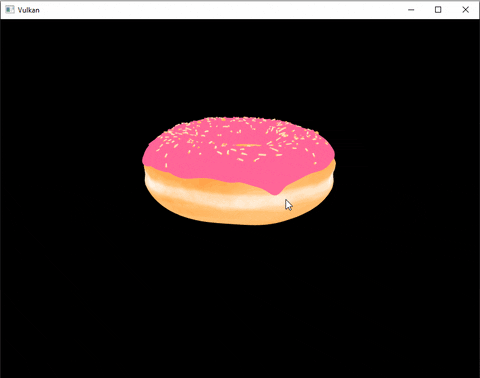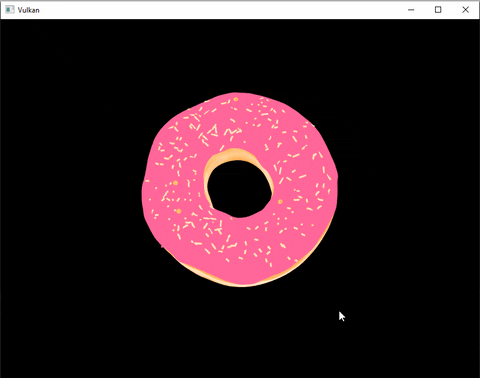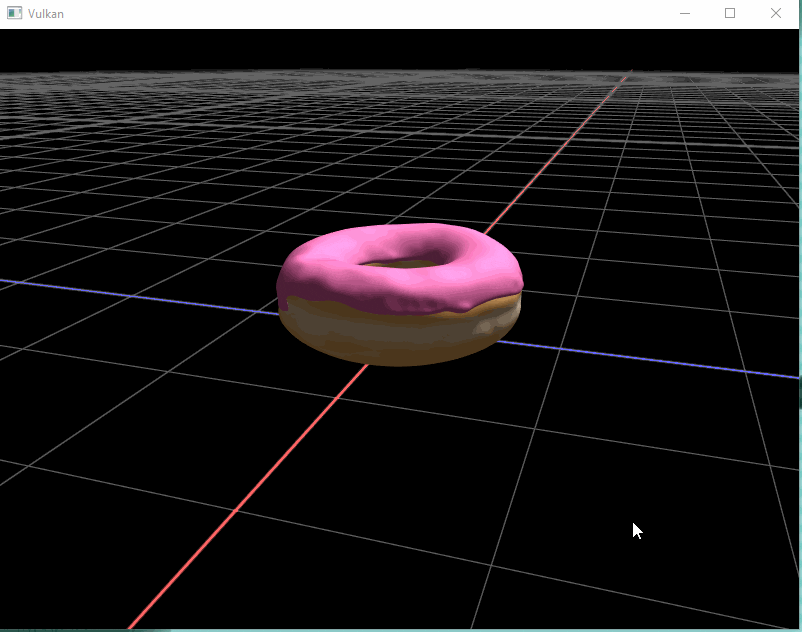# How to implement a simple Arcball Camera.

Keywords: Camera, glm, Rendering, Vulkan, C++, Orbit camera, Arcball camera, Rotation, Computer graphics, 3D programming.

I’m currently working on building a physically based real-time renderer in Vulkan and one of the first thing I did after finishing the intro tutorial (aka 2 weeks later ) was to add an interactive camera so that I can look around my imported model.

Since it was not so easy to find a good example on the web, I thought I could share the method I finaly used to do this.

### What’s an Arcball Camera?

Arcball cameras/orbit cameras are a simple type of camera that rotates around a center point.

Essentially, we want the camera to be rotated on a sphere surrounding the object at the center of our scene.

Here’s an example:### How does it work ?

There are multiple way to implement this. In our case, we are going to find the new position of the camera given the x and y delta angles.The main idea is to rotate the camera using a pivot point. Here’s the algorithm:

1. Calculate the amount of rotation in x and y given the mouse movement.
2. Rotate the camera of theta_x radians around the pivot point on the up axis.
3. Using the updated camera position, rotate the camera of theta_y radians around the pivot point on the right axis.

### Implementation

I’m using glm for matrix operations and all the code will be written in C++.

#### Camera class

First, we are going to define a very simple camera class that contains all the elements required for the Arcball camera.

class Camera
{
public:
Camera() = default;

Camera(glm::vec3 eye, glm::vec3 lookat, glm::vec3 upVector)
: m_eye(std::move(eye))
, m_lookAt(std::move(lookat))
, m_upVector(std::move(upVector))
{
UpdateViewMatrix();
}

glm::mat4x4 GetViewMatrix() const { return m_viewMatrix; }
glm::vec3 GetEye() const { return m_eye; }
glm::vec3 GetUpVector() const { return m_upVector; }
glm::vec3 GetLookAt() const { return m_lookAt; }

// Camera forward is -z
glm::vec3 GetViewDir() const { return -glm::transpose(m_viewMatrix); }
glm::vec3 GetRightVector() const { return glm::transpose(m_viewMatrix); }

void SetCameraView(glm::vec3 eye, glm::vec3 lookat, glm::vec3 up)
{
m_eye = std::move(eye);
m_lookAt = std::move(lookat);
m_upVector = std::move(up);
UpdateViewMatrix();
}

void UpdateViewMatrix()
{
// Generate view matrix using the eye, lookAt and up vector
m_viewMatrix = glm::lookAt(m_eye, m_lookAt, m_upVector);
}

private:
glm::mat4x4 m_viewMatrix;
glm::vec3 m_eye; // Camera position in 3D
glm::vec3 m_lookAt; // Point that the camera is looking at
glm::vec3 m_upVector; // Orientation of the camera
};


#### Arcball rotation

Then, in the application update function that is called every frame, we are going to update the position of the camera given the mouse position. In my case, the up vector is $$y = (0, 1, 0)$$ and my lookat point is $$(0, 0, 0)$$.

We also need to handle the case were the camera view direction is aligned with the up axis.

// Get the homogenous position of the camera and pivot point
glm::vec4 position(app->m_camera.GetEye().x, app->m_camera.GetEye().y, app->m_camera.GetEye().z, 1);
glm::vec4 pivot(app->m_camera.GetLookAt().x, app->m_camera.GetLookAt().y, app->m_camera.GetLookAt().z, 1);

// step 1 : Calculate the amount of rotation given the mouse movement.
float deltaAngleX = (2 * M_PI / viewportWidth); // a movement from left to right = 2*PI = 360 deg
float deltaAngleY = (M_PI / viewportHeight);  // a movement from top to bottom = PI = 180 deg
float xAngle = (app->m_lastMousePos.x - xPos) * deltaAngleX;
float yAngle = (app->m_lastMousePos.y - yPos) * deltaAngleY;

// Extra step to handle the problem when the camera direction is the same as the up vector
float cosAngle = dot(app->m_camera.GetViewDir(), app->m_upVector);
if (cosAngle * sgn(yDeltaAngle) > 0.99f)
yDeltaAngle = 0;

// step 2: Rotate the camera around the pivot point on the first axis.
glm::mat4x4 rotationMatrixX(1.0f);
rotationMatrixX = glm::rotate(rotationMatrixX, xAngle, app->m_upVector);
position = (rotationMatrixX * (position - pivot)) + pivot;

// step 3: Rotate the camera around the pivot point on the second axis.
glm::mat4x4 rotationMatrixY(1.0f);
rotationMatrixY = glm::rotate(rotationMatrixY, yAngle, app->m_camera.GetRightVector());
glm::vec3 finalPosition = (rotationMatrixY * (position - pivot)) + pivot;

// Update the camera view (we keep the same lookat and the same up vector)
app->m_camera.SetCameraView(finalPosition, app->m_camera.GetLookAt(), app->m_upVector);

// Update the mouse position for the next rotation
app->m_lastMousePos.x = xPos;
app->m_lastMousePos.y = yPos;

###### Final Result

Tadaa ! We can now look around this beautiful donut. (I also added a grid and basic lighting by the time I finished this post)